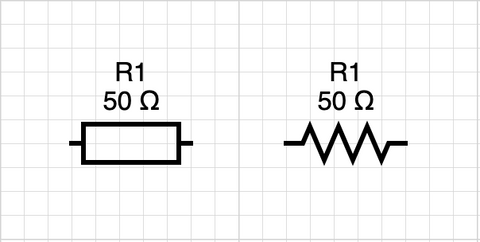# What are resistors? – Understanding Circuit Building Components

As you dive deeper into electronics, it is important to become familiar with the essential components found in many circuits.

Resistors are one of the building blocks engineers get to know like the back of their hand. Learning about resistors will help you grow your skills and tackle bigger, more exciting projects.

## What does a resistor do?

A resistor is a circuit component that does exactly what its name suggests: Resists. Adding a resistor to a circuit reduces the amount of electric current that can pass through the circuit.

Nearly every circuit you encounter uses a resistor in some way. For example, LED circuits, like the ones in our Blink and Hue kits, use current limiting resistors to protect the components from being overpowered by current and burning out! Resistors are also used to control the brightness of the LEDs.

Beyond the basic limiting of current, resistors can be used in more complex and clever applications. Some electronic components require a specific amount of voltage. Adding resistors to a circuit can reduce the voltage used by these other components.

Understanding how a resistor will impact the circuit as a whole is explained by a nifty scientific law called Ohm’s Law.

Resistors are also commonly combined with capacitors, which store energy. These are often referred to as RC circuits and can work in tandem to control frequency filters, current supply, and various other applications.

## What does it look like on a schematic?

Resistors are represented by “squiggly” lines or by empty rectangles depending on the type of diagram you are reading.Next to the resistor symbol on the schematic, there will be either a number or a variable. If it is a variable, it will correspond to a number somewhere else in the documentation. That number indicates the resistance in Ohms(Ω) for that resistor.

## What do the colors mean on a resistor?

The color bands on a resistor indicate the resistance value in Ohms of that specific resistor. It is important to learn how to decode resistor values from the color bands.

To read the resistor value, identify what side has a gap between bands. The side opposite of this is where you will start reading the bands.

The first two stripes indicate the first two digits in the Ohm(Ω) resistance value (see the chart below). Then, the next stripe refers to the multiplier (how many zeros) follow the value.By following this system, you can calculate resistor values easily and clearly. A red, green, red pattern shows a 2500Ω value, and so on.

For resistors with four bands and above, different meanings come into play. The fourth band may indicate higher Ohm values, tolerance, temperature coefficients, fail rates, and more. This can be calculated using a basic resistor chart or a resistor calculator.

## Do resistors have polarity? Does the direction of a resistor matter?

No! Unlike many other components, resistors do not have polarity, meaning you can install them in either direction on a circuit. Also, it does not matter if you are using a through-hole part or surface mount part–the principles and functions of resistors are the same!

## How do I add a resistor to a circuit?

Adding a resistor to your circuit comes down to reading a circuit board correctly and soldering in the correct place.

In the Blink and Hue Learn to Solder Kits, the online instructions provide detailed information about installing the resistors so that anyone without prior experience can understand how to do it. Plus, you get great experience learning how to solder resistor components!